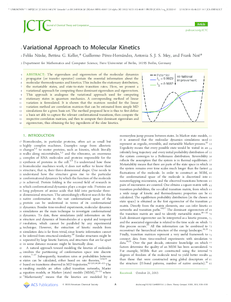Repository: Freie Universität Berlin, Math Department

# Variational Approach to Molecular Kinetics

Nüske, F. and Keller, B. and Pérez-Hernández, G. and Mey, A.S.J.S. and Noé, F. (2014) Variational Approach to Molecular Kinetics. J. Chem. Theory Comput., 10 . pp. 1739-1752.Preview
PDF - Accepted Version
2MB

## Abstract

The eigenvalues and eigenvectors of the molecular dynamics propagator (or transfer operator) contain the essential information about the molecular thermodynamics and kinetics. This includes the stationary distribution, the metastable states, and state-to-state transition rates. Here, we present a variational approach for computing these dominant eigenvalues and eigenvectors. This approach is analogous the variational approach used for computing stationary states in quantum mechanics. A corresponding method of linear variation is formulated. It is shown that the matrices needed for the linear variation method are correlation matrices that can be estimated from simple MD simulations for a given basis set. The method proposed here is thus to first define a basis set able to capture the relevant conformational transitions, then compute the respective correlation matrices, and then to compute their dominant eigenvalues and eigenvectors, thus obtaining the key ingredients of the slow kinetics.

Item Type: Article Physical SciencesMathematical and Computer Sciences Department of Mathematics and Computer Science > Institute of Mathematics > Comp. Molecular Biology 1388 BioComp Admin 01 Mar 2014 17:31 03 Mar 2017 14:41

Repository Staff Only: item control page